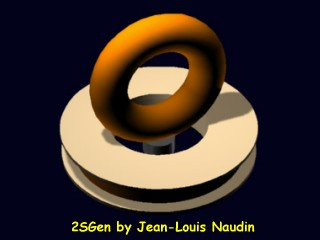S2Gen Episode 7: Measuring the ratio between the Demagnetization energy and the Magnetization energy
created on february 15, 2009 - JLN Labs - Last update march 3, 2010
Toutes les informations et schémas sont publiés gratuitement ( freeware ) et sont destinés à un usage personnel et non commercial
All informations and diagrams are published freely (freeware) and are intended for a private use and a non commercial use.

In the previous test (episode 6), we have seen that the collected demagnetization energy of the core is greater, in some cases, than the magnetization energy spent, according to the Nikolay E. Zaev paper. The purpose of this new test is to measure the ratio between the demagnetization energy and the magnetization energy, this will give us a very good idea of the perfomances of the 2SGen device.

To conduct this test, I have used the 2SGen v5 setup with the neodymium magnet placed in the middle of the toroïdal coil, see below.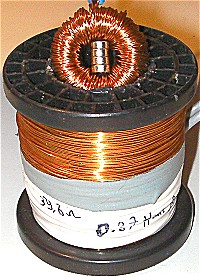A pulse generator has been connected to the 2SGen MosFet driver and the squared pulse frequency has been set to 990 Hz.

Two separate circuits have been connected to the output coil. The first one has been used as the load for the magnetization phase and the second one has been used as the load for the demagnetization phase of the core. Each circuit is composed of a 10 kOhm resistor as the load, one high speed diode BYW98/200 as the rectifier and one 22 µF capacitor as the regulator. The voltages are measured across each load with a digital voltmeter, a digital scope has also been used to check if the measured voltages across the loads are pure DC. So, by this way, it is very simple to measure the power ratio l between the demagnetization phase (dem) and the magnetization phase (mag).

This is given by the formula : l = ((Vdem^2)/10000) / ((Vmag^2)/10000) and thus, the l factor gives a good idea of the performances of the 2SGen.

Of course, to get a true 2SGen unit working in closed loop, the driver circuit must be optimized and needs to overcome the hystesis losses and the Joule losses. Nevertheless, the physic phenomenon here is very interesting and worth to be deeply explored, the 2SGen is a very promising device...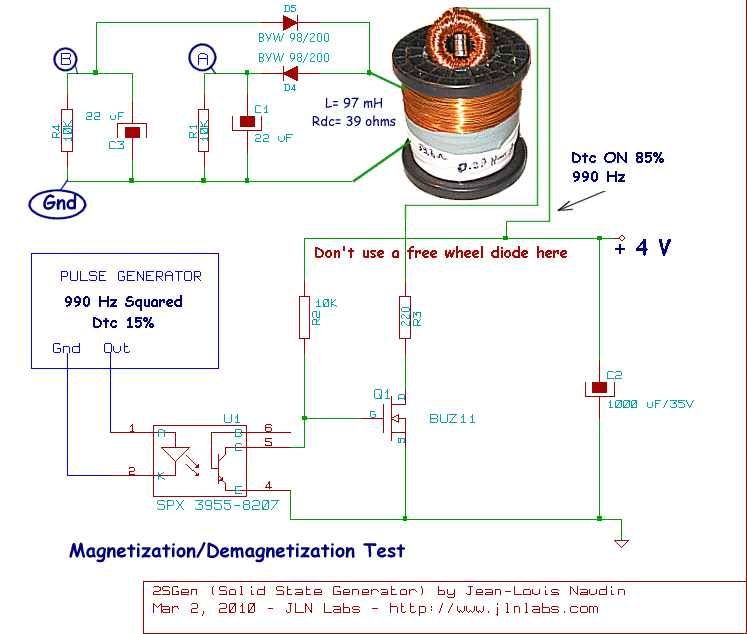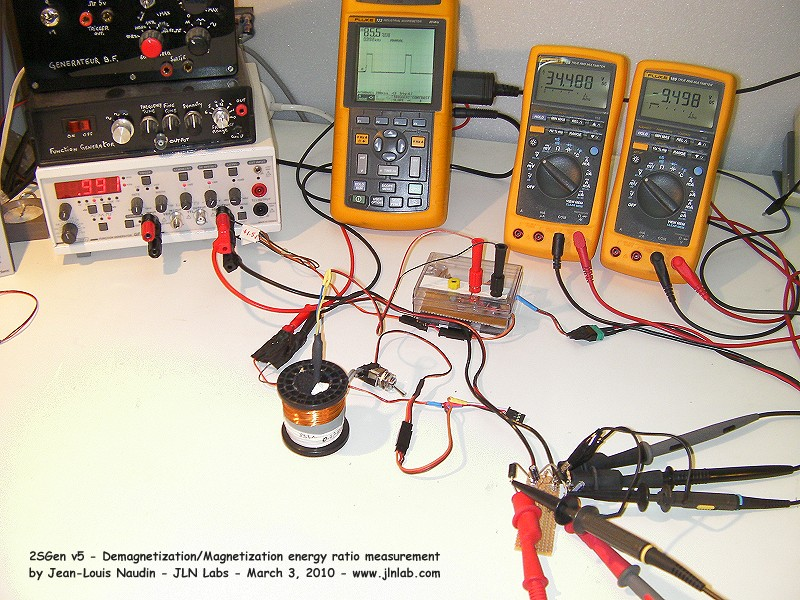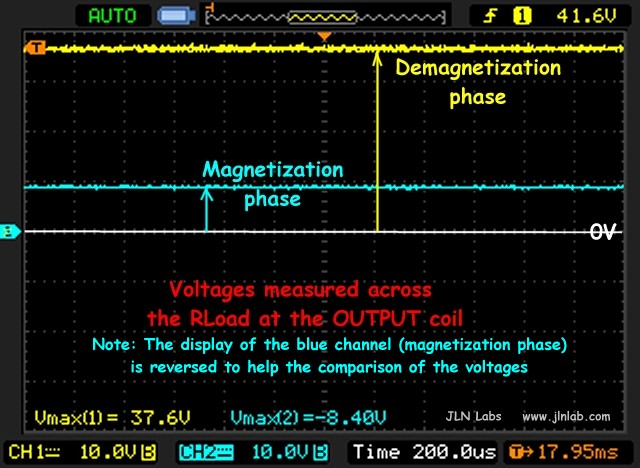As you may see in the scope picture above, the voltages measured across the loads are pure DC (yellow and light blue curves)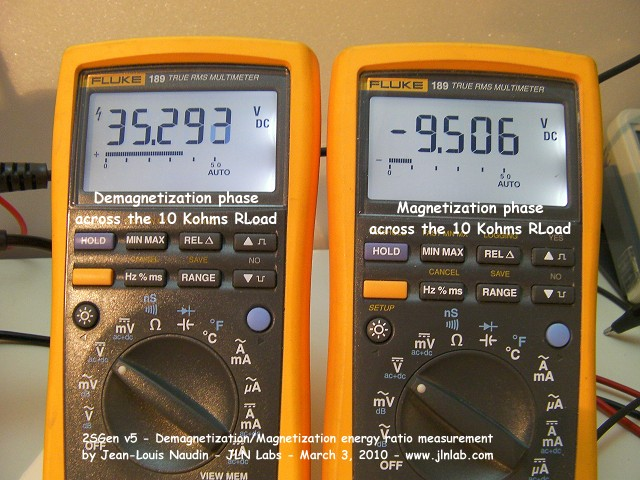l
= ((Vdem^2)/10000) / ((Vmag^2)/10000) = ((35.2^2)/10000) / ((9.5^2)/10000) = 13.7
It is interesting to notice that N. Zaev has found a
l = 16.3 with a Permalloy 81 NM core (look at here)

Look at the full video of this test below:

Here an interesting paper to read :"Inductive conversion of heat environmental energy to electrical energy" from Nikolay E. Zaev - New Energy Technologies Issue #1 (4) Jan-feb 2002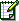Email : JNaudin509@aol.com